### Home > CALC > Chapter 5 > Lesson 5.1.1 > Problem5-10

5-10.
1. Examine the following integrals. Consider the multiple tools available for evaluating integrals and use the best strategy for each. After evaluating the integral, write a short description of your method. Homework Help ✎

1.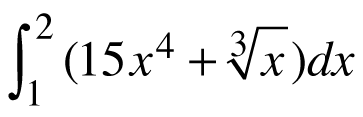2.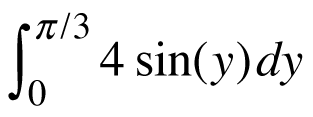3.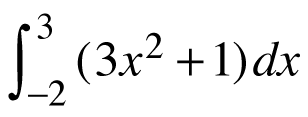4.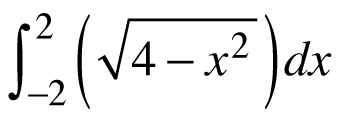5.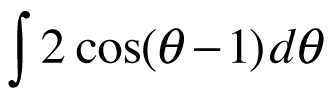6.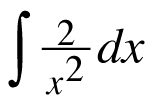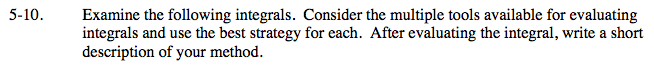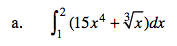Before integrating, rewrite the integrand with a fractional exponent.

$\int_1^2(15x^4+x^{1/3})dx=3x^5+\frac{3}{4}x^{4/3}\Big|_1^2=?$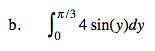$\int\sin(x)dx=-\cos(x)+C$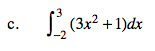Undo the Power Rule.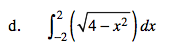Do NOT use a calculator!
This function is a graph of a semicircle.
Calculate the area using geometry.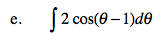Determine the antiderivative by shifting and stretching the antiderivative of y = cos(x).

2sin(θ − 1) + C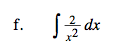Before integrating, rewrite the integrand with a negative exponent.